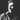# Functional programming with Ramda.js

September 05, 2015

JavaScript is one of the most dynamic, flexible and powerful programming languages. It supports multiple programming paradigms - imperative, object (prototype) oriented, scripting, and functional.
Let’ see what JavaScript has common with functional programming languages:

• First-class functions - functions are objects themselves
• Anonymous functions - functions can be unnamed and nested
• Closures - functions that refer to independent variables, that were created in other scope
• Recursion - function can call itself

By the way, there are some significant differences:

• Immutability - objects, functions in JavaScript can be modified after creation
• Pure functions - JavaScript functions often depends on outside scope, and it’s hard to create function that always returns the same result with given same parameters
• Strong (and static) typing - JavaScript allows use a value of one type as if it were a value of another type, and has not static typing system

JavaScript ecosystem has great tools for advanced use of functional programming features, such as Underscore and Lodash - most popular toolkit libraries. But if you want use library, that was specifically designed for functional programming, you may have to look at Ramda.js .

Ramda.js has some distinguishing features:

• It’s designed in pure functional style, with immutability and side-effects free functions, that helps you to write simple and effective code.
• Parameters in functions have the same order, with key params at first and data at last
• Ramda.js functions are automatically curried, that allows you to easily build new functions from old ones

To show examples of Ramda.js using, i will use io.js 3.2 and Babel, so let’s create new .js file:

``````#!/usr/bin/env babel-node

import R from "ramda"``````

Ramda.js API has some general use functions:

``````// Typing
let str = "test"
R.is(String, str) //=> true
// The same with currying
R.is(String)(str) //=> true
let isString = R.is(String)
isString("string") //=> true
R.type(isString) //=> String

// Math
R.mean([2, 3, 7]) //=> 4
R.sum(R.range(1, 5)) //=> 10

// Logic
R.and(true, false) //=> false
R.and([])(0) //=> 0
R.not(1) //=> false
R.both(isString, R.is(String))(str) // => true``````

Like the Underscore and Lodash, Ramda has collection helper functions:

``````// Lists
let animals = [
{
name: "goose",
type: "bird",
color: "white",
},
{
name: "parrot",
type: "bird",
color: "yellow",
},
{
name: "cat",
type: "mammal",
color: "grey",
},
]

R.map(animal => animal.color + " " + animal.name, animals) //=> [ 'white goose', 'yellow parrot', 'grey cat' ]
R.last(animals).name //=> cat
R.uniq(R.pluck("type", animals)) //=> [ 'bird', 'mammal' ]
R.length(R.filter(animal => animal.type === "bird", animals)) //=> 2``````

And object helpers too:

``````// Objects
let cat = {
type: "animal",
subclass: "mammal",
binomialName: "Felis catus",
}
R.assoc("status", "domesticated", cat).status //=> domesticated
R.dissoc("binomialName", cat).binomialName //=> undefined
R.keys(cat) //=> [ 'type', 'subclass', 'binomialName' ]
R.has("type", cat) //=> true
R.prop("type", cat) //=> animal
R.values(cat) //=> [ 'animal', 'mammal', 'felis catus' ]

// Object transformation
let transformations = {
type: R.toUpper,
binomialName: R.toLower,
}
R.evolve(transformations, cat).type // => ANIMAL``````

But key point of Ramda.js is functions. Ramda allows you to easily compose multiple functions in different orders:

``````// Compose and pipe
R.join(' and ', R.uniq(R.map(R.toUpper)(R.pluck('type', animals)))); //=> BIRD and MAMMAL
// Performs right-to-left function composition
R.compose(
R.join(' and '),
R.uniq,
R.map(R.toUpper),
R.pluck('type')
)(animals); //=> BIRD and MAMMAL
// Performs left-to-right function composition
R.pipe(
R.pluck('type')
R.map(R.toUpper),
R.uniq,
R.join(' and ')
)(animals); //=> BIRD and MAMMAL``````

Another power of Ramda is currying. Currying is the process of translating evaluation of function that takes multiple parameters in evaluating a sequence of functions, each with one argument.

``````let tripleMultiply = (a, b, c) => a _ b _ c;
tripleMultiply(3, 9, 2); //=> 54
tripleMultiply(3, 9)(2); //=> TypeError: tripleMultiply(..) is not a function
let curriedMultiply = R.curry(tripleMultiply);
curriedMultiply(3, 9)(2); //=> 54
curriedMultiply(3)(9)(2); //=> 54``````

Pattern matching is also available through R.cond. That allows you to check sequence of conditions to match different patterns:

``````let checkNumber = R.cond([
[R.is(Number), R.identity],
[R.is(String), parseInt],
[R.is(Boolean), Number],
[R.isEmpty, R.always(0)],
[R.T, R.always(NaN)],
])
checkNumber(100500) //=> 100500
checkNumber("146%") //=> 146
checkNumber("Hodor") //=> NaN
checkNumber(true) //=> 1
checkNumber(false) //=> 0
checkNumber([]) //=> 0
checkNumber(["test"]) //=> NaN``````

Ramda.js is one of the best functional programming libraries that exists in JavaScript ecosystem. It can completely replace Underscore, Lodash in your project with own object, lists and others helpers. Immutability, currying and composing allows you to write both efficient and simple code in pure functional style.

Tags:Aleksei Kuznetsov

Functional programming, software architecture, DevOps

Salam